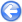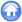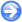### 4.2PASS: Convergence for a simple periodic problem

Author
Stéphane Popinet
Command
sh periodic.sh periodic.gfs
Version
0.6.4
Required files
periodic.sh r0.ref r1.ref r2.ref
Running time
47 minutes 45 seconds

This is one of the test cases presented in Popinet . Following Minion  and Almgren et al. , this convergence test illustrates the second-order accuracy of Gerris for flows without solid boundaries. This problem uses a square unit domain with periodic boundary conditions in both directions. The initial conditions are taken as

 u(x,y) = 1−2cos(2π x)sin(2π y), v(x,y) = 1+2sin(2π x)cos(2π y).

The exact solution of the Euler equations for these initial conditions is

 u(x,y,t) = 1−2cos(2π(x−t))sin(2π(y−t)), v(x,y,t) = 1+2sin(2π(x−t))cos(2π(y−t)), p(x,y,t) = −cos(4π(x−t))−cos(4π(y−t)).

As in  nine runs are performed on grids with L=5,6 and 7 levels of refinement (labelled “uniform”) and with one (labelled r=1) or two (labelled r=2) additional levels added only within the square defined by the points (−0.25,−0.25) and (0.25,0.25). The length of the run for each case is 0.5, the CFL number is 0.75. For each run both the L2 and L norms of the error in the x-component of the velocity is computed. Table 3 gives the errors and order of convergence obtained.

Close to second-order convergence is obtained (asymptotically in L) for the L2 and L norms. The values obtained are comparable to that in [29, 1].

 Table 3: Errors and convergence orders in the x-component of the velocity for a simple periodic problem. The reference solution values are given in blue.
 L2 L=5 O2 L=6 O2 L=7 Uniform 8.27e-03 2.84 1.15e-03 2.48 2.07e-04 8.27e-03 2.84 1.15e-03 2.48 2.07e-04 r=1 8.35e-03 2.29 1.70e-03 2.11 3.96e-04 8.35e-03 2.29 1.70e-03 2.11 3.96e-04 r=2 1.06e-02 2.08 2.50e-03 2.00 6.24e-04 1.06e-02 2.08 2.50e-03 2.00 6.24e-04 L∞ L=5 O∞ L=6 O∞ L=7 Uniform 1.97e-02 2.66 3.10e-03 2.61 5.08e-04 1.97e-02 2.66 3.10e-03 2.61 5.08e-04 r=1 2.20e-02 2.21 4.77e-03 2.06 1.14e-03 2.20e-02 2.21 4.77e-03 2.06 1.14e-03 r=2 2.82e-02 2.11 6.54e-03 1.99 1.65e-03 2.82e-02 2.11 6.54e-03 1.99 1.65e-03